## 4.2.1 Device Structure and Properties

Fig. 4.8 shows the structure of a MAGFET. The printed gate length is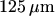, the oxide thickness is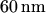and the device width is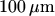. The drain is split into two contacts with a distance of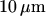from each other. If no magnetic field is applied and the bias polarization at the drains are the same, the device behaves exactly like a MOSFET. However, if a magnetic field is present (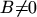) the carriers in the channel are deflected as a result of the Lorentz force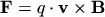(4.1)

where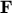denotes the Lorentz force,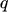is the charge of the carriers, and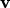is the carrier velocity. This charge deflection results in a perturbed balance of the current flow in the channel area and thus in different currents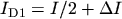and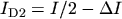measured in the two drains.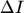is a function of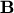and the geometry of the device . The relative sensitivity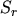%of a MAGFET is defined as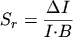(4.2)

where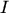denotes the total current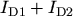. In  the sensitivity is obtained as a function of several geometric parameters, such as the distance of the two drains, the channel length, the width of the source contact, and the gate overlap, but also the gate and the drain voltages. Moreover, the sensitivity is dramatically increased for low temperatures [K7].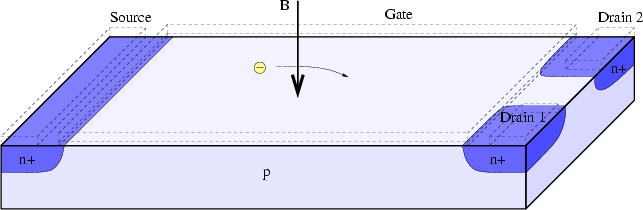Robert Klima 2003-02-06